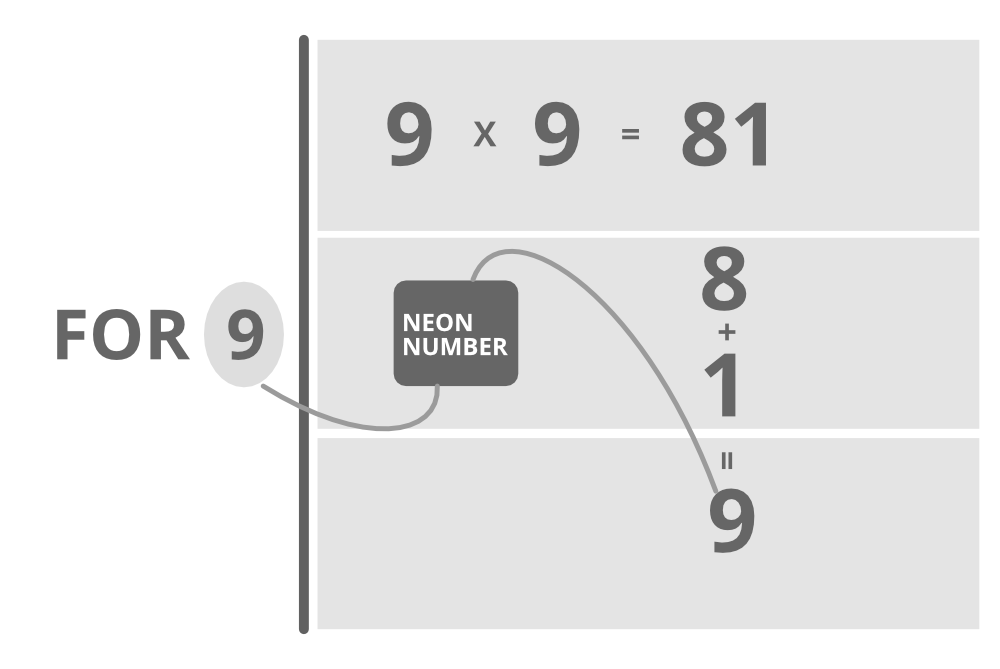Open in App
Not now

# Java Program to Check If a Number is Neon Number or Not

• Difficulty Level : Easy
• Last Updated : 15 Oct, 2021

A neon number is a number where the sum of digits of the square of the number is equal to the number. The task is to check and print neon numbers in a range.Illustration:

```Case 1:

Input  : 9
Output : Given number  9 is Neon number

Explanation : square of 9=9*9=81;
sum of digit of square : 8+1=9(which is equal to given number)

Case 2:

Input : 8
Output : Given number is not a Neon number

Explanation : square of 8=8*8=64
sum of digit of square : 6+4=10(which is not equal to given number)```

Algorithm :

1. First, find the square of the given number.
2. Find the sum of the digit of the square by using a loop.

3. The condition checksum is equal to the given number
1. Return true
2. Else return false.
```Pseudo code : Square =n*n;
while(square>0)
{
int r=square%10;
sum+=r;
square=square/10;
}```

Example:

## Java

 `// Java Program to Check If a Number is Neon number or not` `// Importing java input/output library``import` `java.io.*;` `class` `GFG {` `    ``// Method to check whether number is neon or not``    ``// Boolean type``    ``public` `static` `boolean` `checkNeon(``int` `n)``    ``{``        ``// squaring the number to be checked``        ``int` `square = n * n;` `        ``// Initializing current sum to 0``        ``int` `sum = ``0``;` `        ``// If product is positive``        ``while` `(square > ``0``) {` `            ``// Step 1: Find remainder``            ``int` `r = square % ``10``;` `            ``// Add remainder to the current sum``            ``sum += r;` `            ``// Drop last digit of the product``            ``// and store the number``            ``square = square / ``10``;``        ``}` `        ``// Condition check``        ``// Sum of digits of number obtained is``        ``// equal to original number``        ``if` `(sum == n)` `            ``// number is neon``            ``return` `true``;``        ``else` `            ``// number is not neon``            ``return` `false``;``    ``}` `    ``// Main driver method``    ``public` `static` `void` `main(String[] args)``    ``{``        ``// Custom input``        ``int` `n = ``9``;` `        ``// Calling above function to check custom number or``        ``// if user entered number via Scanner class``        ``if` `(checkNeon(n))` `            ``// Print number considered is neon``            ``System.out.println(``"Given number "` `+ n``                               ``+ ``" is Neon number"``);``        ``else` `            ``// Print number considered is not neon``            ``System.out.println(``"Given number "` `+ n``                               ``+ ``" is not a Neon number"``);``    ``}``}`

Output

`Given number 9 is Neon number`

Time Complexity: O(l) where l is the number of the digit in the square of the given number

My Personal Notes arrow_drop_up